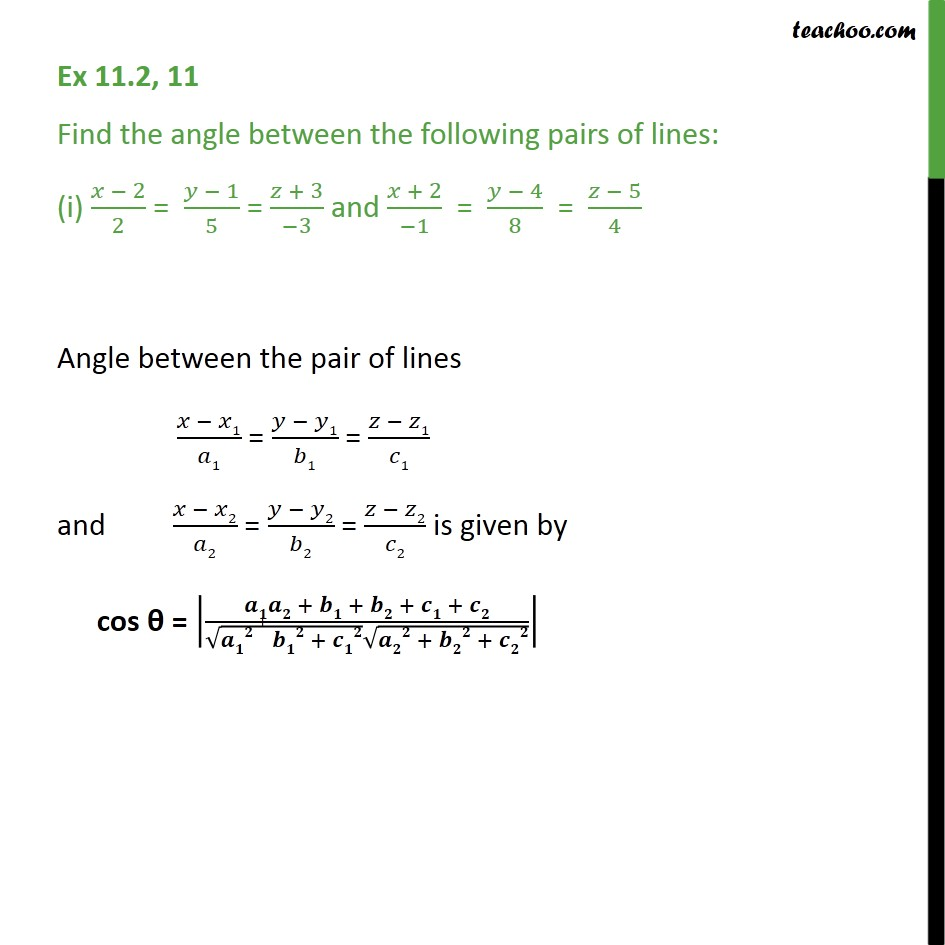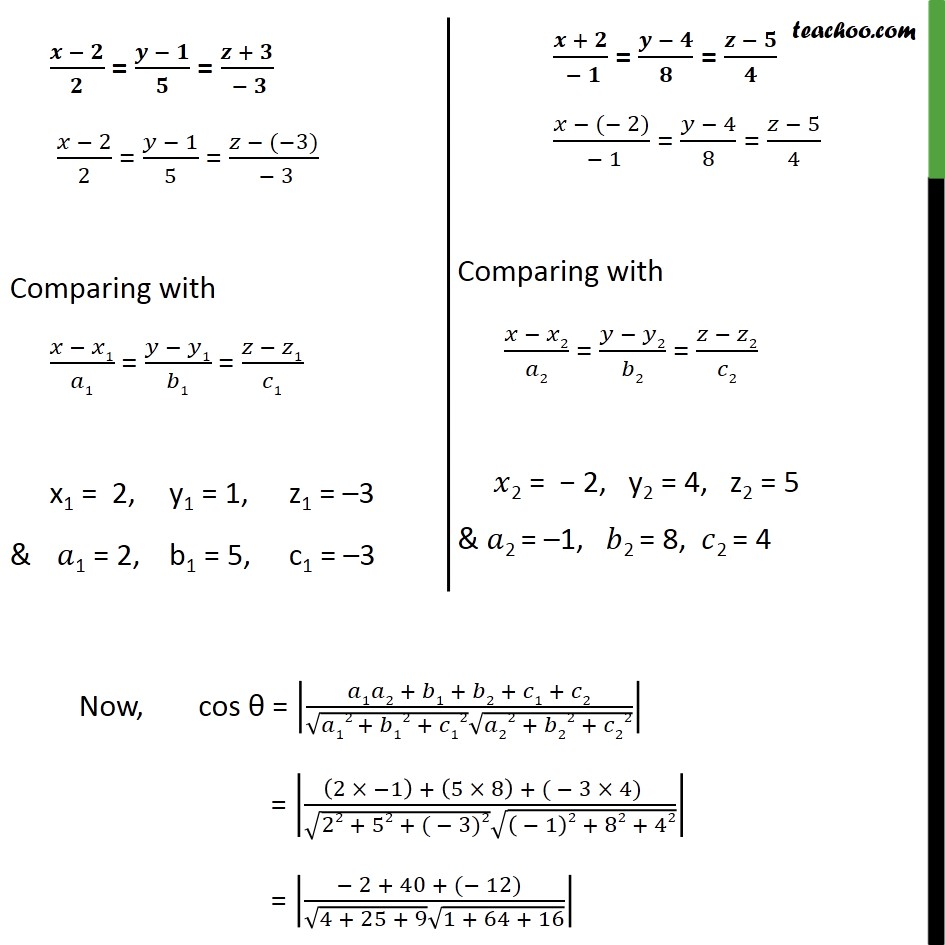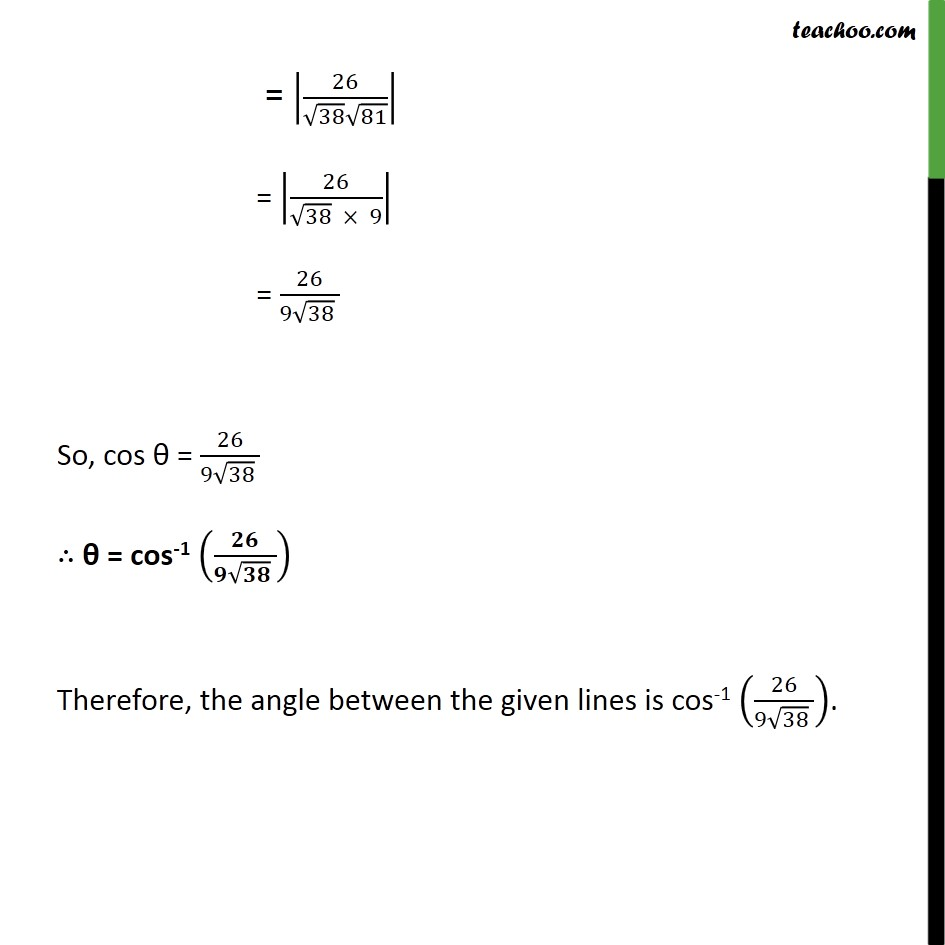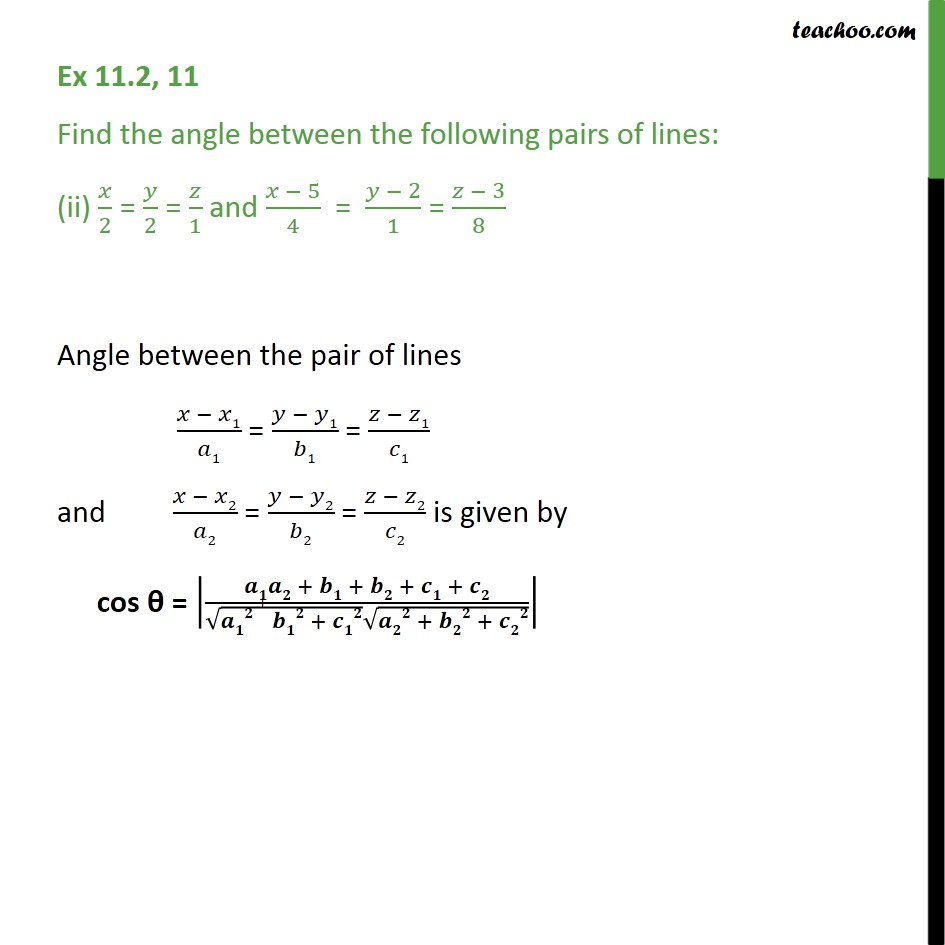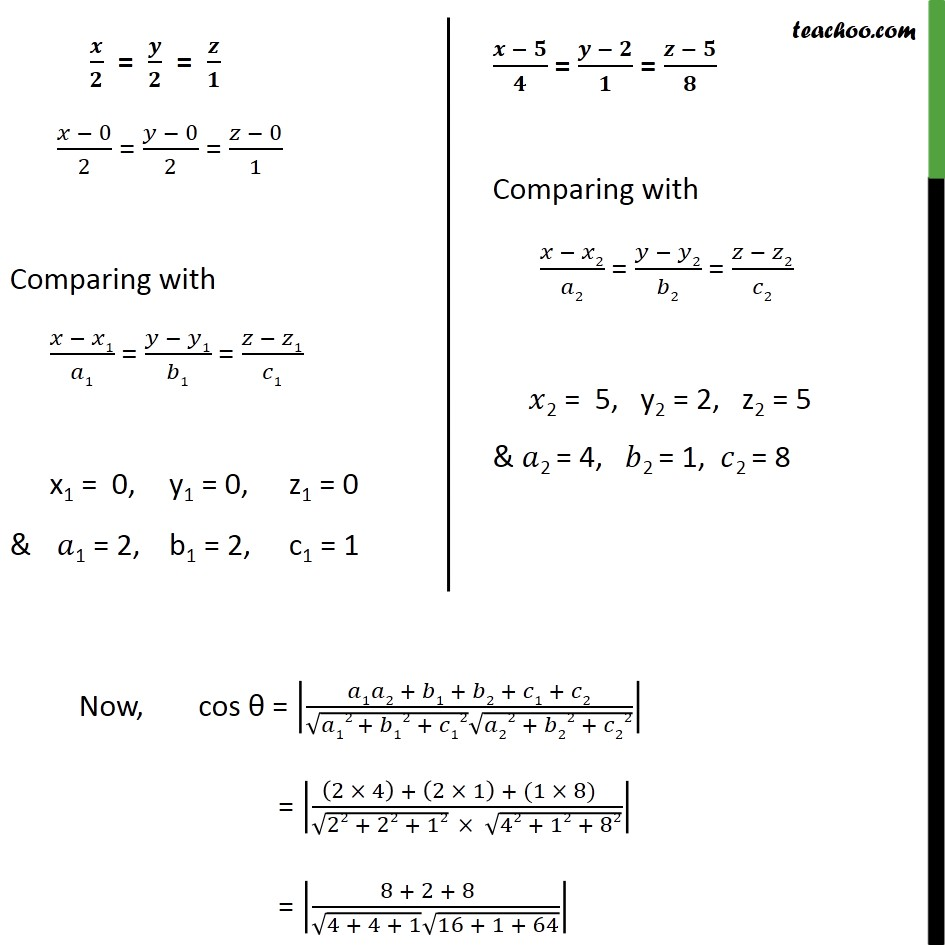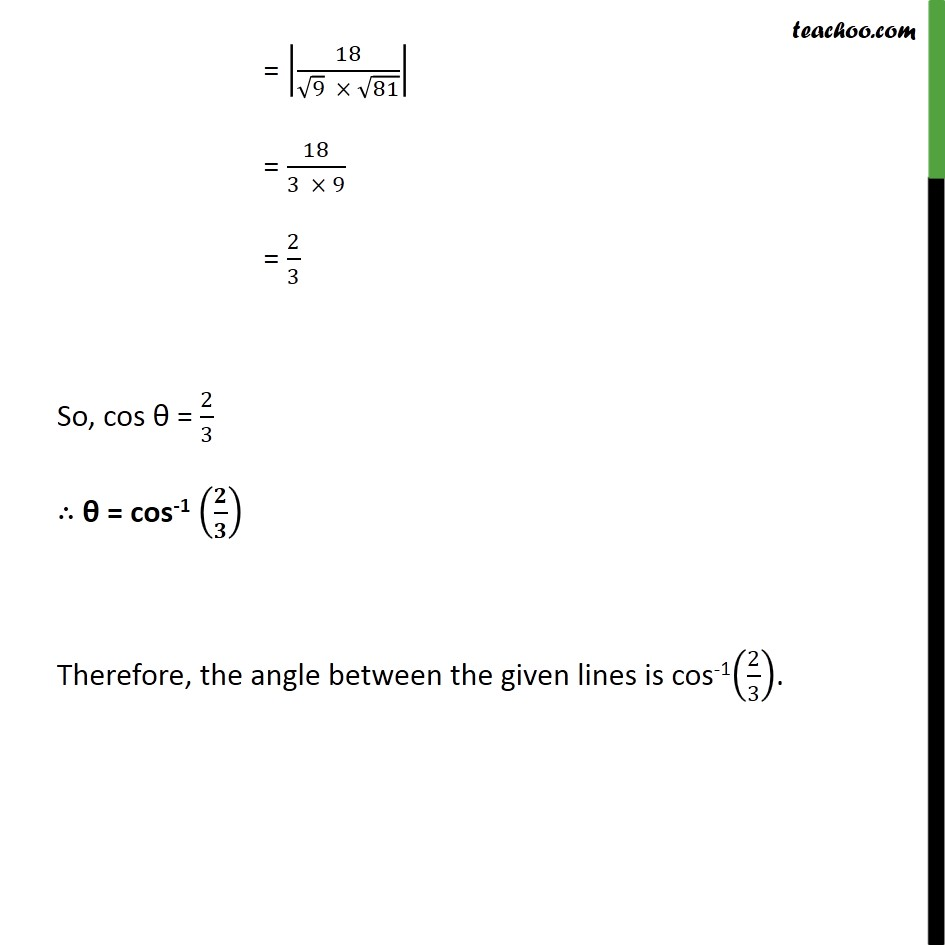1. Chapter 11 Class 12 Three Dimensional Geometry
2. Serial order wise
3. Ex 11.2

Transcript

Ex 11.2, 11 Find the angle between the following pairs of lines: (i) 𝑥 − 2﷮2﷯ = 𝑦 − 1﷮5﷯ = 𝑧 + 3﷮−3﷯ and 𝑥 + 2﷮−1﷯ = 𝑦 − 4﷮8﷯ = 𝑧 − 5﷮4﷯ Angle between the pair of lines 𝑥 − 𝑥1﷮𝑎1﷯ = 𝑦 − 𝑦1﷮𝑏1﷯ = 𝑧 − 𝑧1﷮𝑐1﷯ and 𝑥 − 𝑥2﷮𝑎2﷯ = 𝑦 − 𝑦2﷮𝑏2﷯ = 𝑧 − 𝑧2﷮𝑐2﷯ is given by cos θ = 𝒂𝟏𝒂𝟐 + 𝒃𝟏 + 𝒃𝟐 + 𝒄𝟏 + 𝒄𝟐﷮ ﷮𝒂𝟏𝟐 + 𝒃𝟏𝟐 + 𝒄𝟏𝟐﷯ ﷮𝒂𝟐𝟐 + 𝒃𝟐𝟐 + 𝒄𝟐𝟐﷯﷯﷯ = 26﷮ ﷮38﷯ ﷮81﷯﷯﷯ = 26﷮ ﷮38﷯ × 9﷯﷯ = 26﷮9 ﷮38﷯ ﷯ So, cos θ = 26﷮9 ﷮38﷯ ﷯ ∴ θ = cos-1 𝟐𝟔﷮𝟗 ﷮𝟑𝟖﷯ ﷯﷯ Therefore, the angle between the given lines is cos-1 26﷮9 ﷮38﷯ ﷯﷯. Ex 11.2, 11 Find the angle between the following pairs of lines: (ii) 𝑥﷮2﷯ = 𝑦﷮2﷯ = 𝑧﷮1﷯ and 𝑥 − 5﷮4﷯ = 𝑦 − 2﷮1﷯ = 𝑧 − 3﷮8﷯ Angle between the pair of lines 𝑥 − 𝑥1﷮𝑎1﷯ = 𝑦 − 𝑦1﷮𝑏1﷯ = 𝑧 − 𝑧1﷮𝑐1﷯ and 𝑥 − 𝑥2﷮𝑎2﷯ = 𝑦 − 𝑦2﷮𝑏2﷯ = 𝑧 − 𝑧2﷮𝑐2﷯ is given by cos θ = 𝒂𝟏𝒂𝟐 + 𝒃𝟏 + 𝒃𝟐 + 𝒄𝟏 + 𝒄𝟐﷮ ﷮𝒂𝟏𝟐 + 𝒃𝟏𝟐 + 𝒄𝟏𝟐﷯ ﷮𝒂𝟐𝟐 + 𝒃𝟐𝟐 + 𝒄𝟐𝟐﷯﷯﷯ = 18﷮ ﷮9﷯ × ﷮81﷯﷯﷯ = 18﷮3 × 9﷯ = 2﷮3﷯ So, cos θ = 2﷮3﷯ ∴ θ = cos-1 𝟐﷮𝟑﷯﷯ Therefore, the angle between the given lines is cos-1 2﷮3﷯﷯.

Ex 11.2